# Building a Climate III¶

This lecture introduces radiative fluxes and the influence of insolation, albedo and greenhouse gases on average planetary temperatures. After this lecture, you should be able to predict average temperature of planets and understand why this is possible.

## Information¶

Learning goals

• Gain quantitative understanding of radiative fluxes in the atmosphere

• Understand influence of insolation and greenhouse gases on mean temperature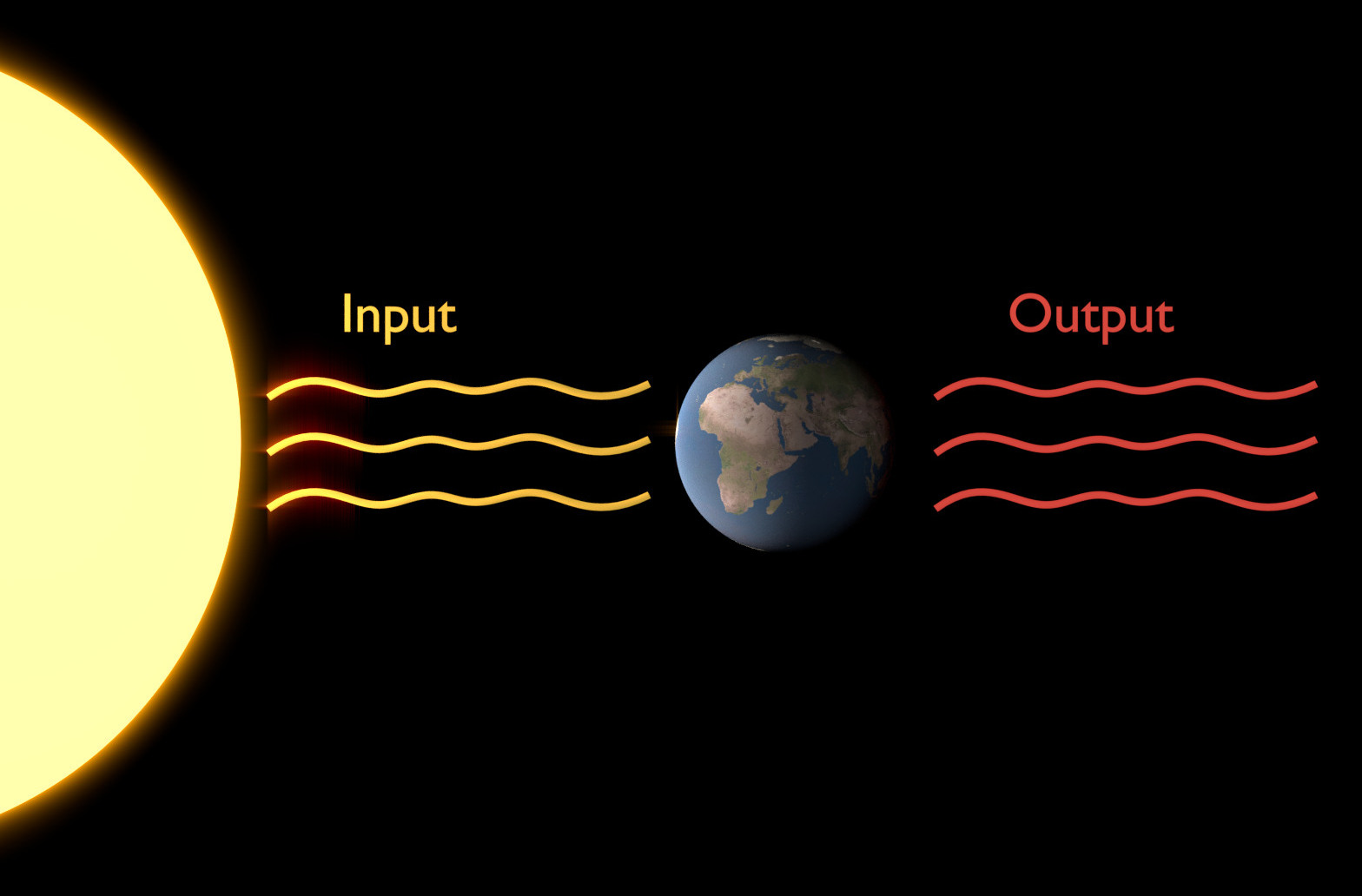The concept of black body radiation is essential in understanding radiative fluxes (the input and output) of Earth. Specifically, it lets us calculate the nature of incoming and outgoing radiation, as well as the mean global temperatures on Earth or any planet if the whole system is in equilibrium. [image: cc-by Sebastian Mutz]

A black body is an idealised opaque, non-reflective object or system that absorbs all incident radiation and re-radiates energy. Black body radiation is the electromagnetic radiation emitted by a black body (that is in thermodynamic equilibrium with its environment). Its spectrum of wavelengths depends only on the body’s temperature. The characteristics of black body radiation are therefore only dependent on the system’s attributes (temperature) rather than the nature of previously absorbed radiation. The concept of black body radiation is central in understanding the important radiative fluxes of the climate system and global mean temperatures on Earth.

### Wien’s Law¶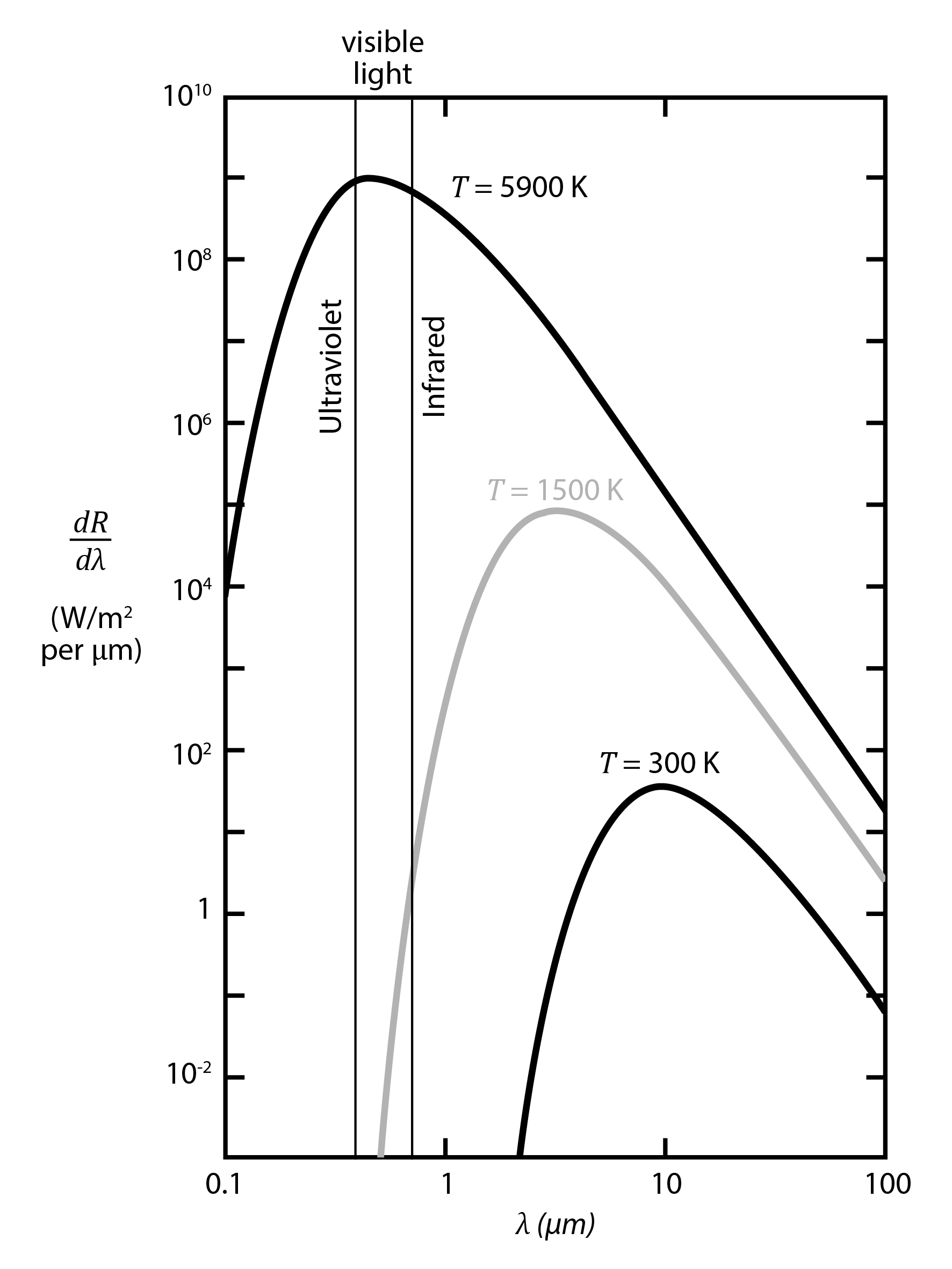An increase in temperature causes higher emission of radiation of all wavelenghts and shifts the radiation peak towards shorter wavelengths. [image: cc-by Lisa Rauschenbach]

In case of black body radiation, an increase in temperature causes higher emission of radiation of all wavelenghts, but esp. for short wavelength radiation. Therefore, the radiation peak on the wavelength spectrum shifts as temperature changes. This is described by Wien’s Law, which states that the emission curve (see figure) peaks at different wavelengths inversely proportional to temperature:

$\begin{equation} \lambda_{max} = \frac {C}{T} \end{equation}$

In the equation above:

• $$\lambda_{max}$$ - the maximum wavelength

• c - proportionality constant; ca. 2.897·10-3

• T - temperature

Two important peaks to remember for the climate system on Earth are:

$\lambda_{max(sun)} \approx 0.49 \mu m$
$\lambda_{max(earth)} \approx 0.96 \mu m$

### Revisiting the Electromagnetic Spectrum¶

Before we move on, let us remind ourselves of the electromagnetic spectrum, the visible spectrum and where the energy in Earth’s climate system fits. Examine the figure below.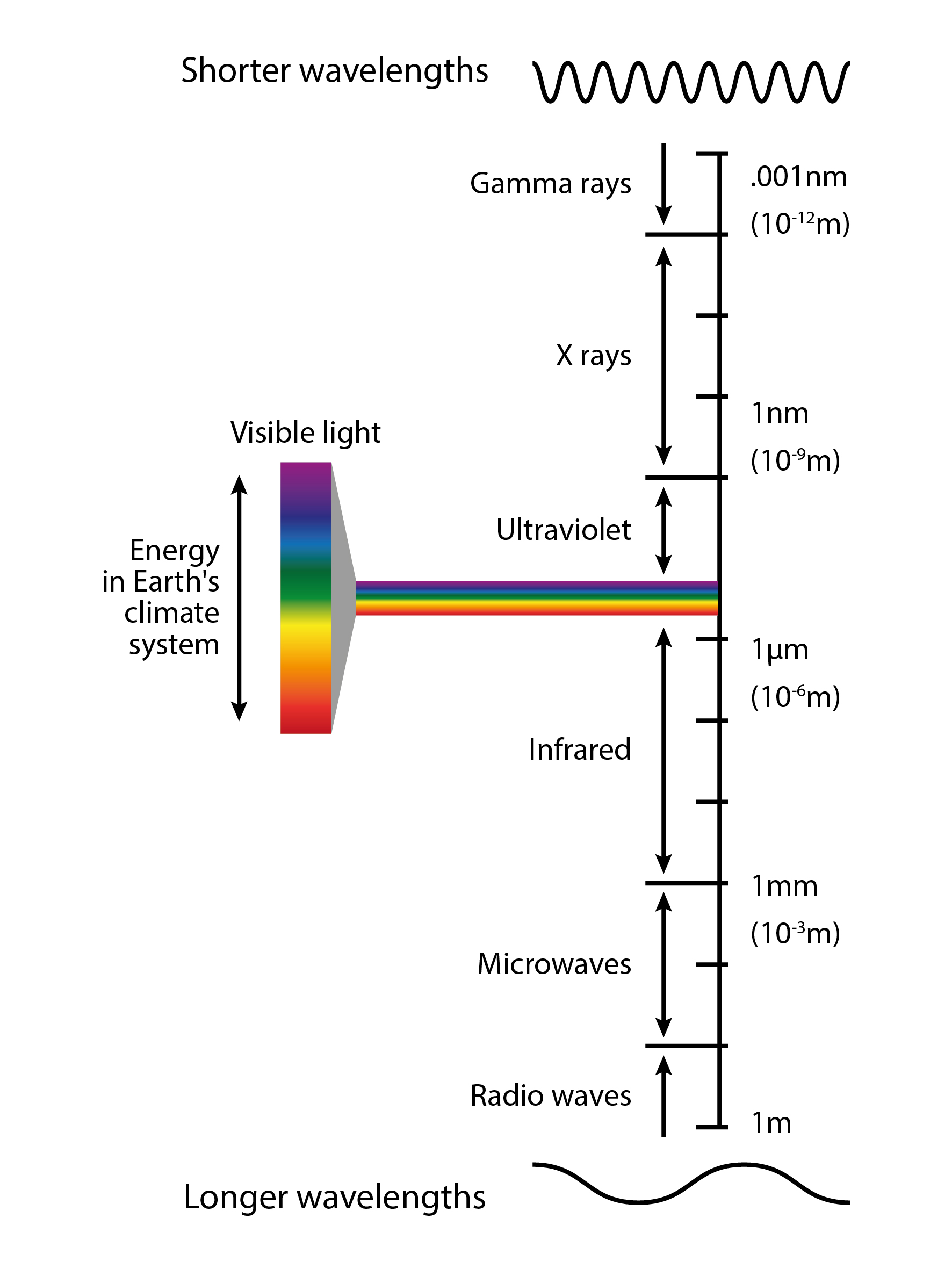The electromagnetic spectrum, visible light and where the energy in Earth’s climate system fits. [image: cc-by Lisa Rauschenbach]

Note

Determine the peak radiation for different temperature black bodies. Repeat this for the sun and Earth. Do your results match the previous figure? Why do you think we are able to see this particular range in the electromagnetic spectrum?

### Stefan-Boltzman Law¶

Stefan-Boltzman Law describes the total energy radiated per unit time per surface area of a black body across all wavelengths. It can be calculated as follows:

$\begin{equation} \frac {power}{area} = \sigma · T^4 \end{equation}$

Sigma ($$\sigma$$ ) is the Stefan-Boltzman constant. $$\sigma = 5.670373 · 10^{-8}Wm^{-2}K^{-4}$$ . We will later make use of this equation to calculate the mean global temperature of Earth.

Note

The sun’s temperature is approximately 5778K. Can you calculate its power output per area? How would you calculate its total power output (not per area)?

## The Solar Constant¶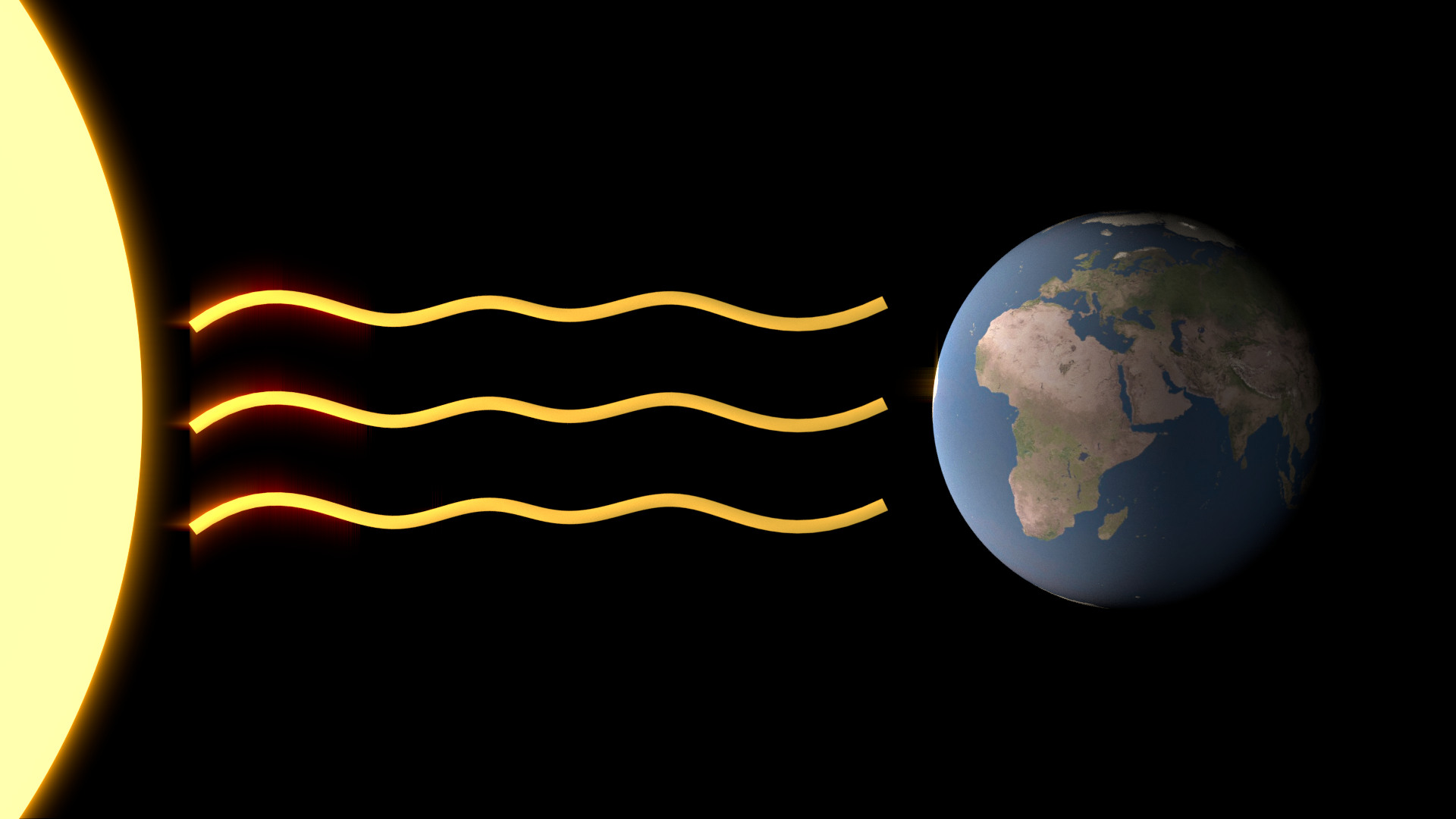The sun fuses 4H to He and releases solar electromagnetic radiation in the process. It’s the main (and only significant) energy input to Earth’s climate system. [image: cc-by Sebastian Mutz]

The solar constant ($$E_0$$ ) is the radiation intensity that hits the outer atmosphere of Earth and thus an important parameter to remember in climatology. $$E_0 \approx 1380Wm^{-2}$$ , but let’s take a step back and see where that number comes from. To unterstand where the number comes from, let’s go back to the source of it all: the sun.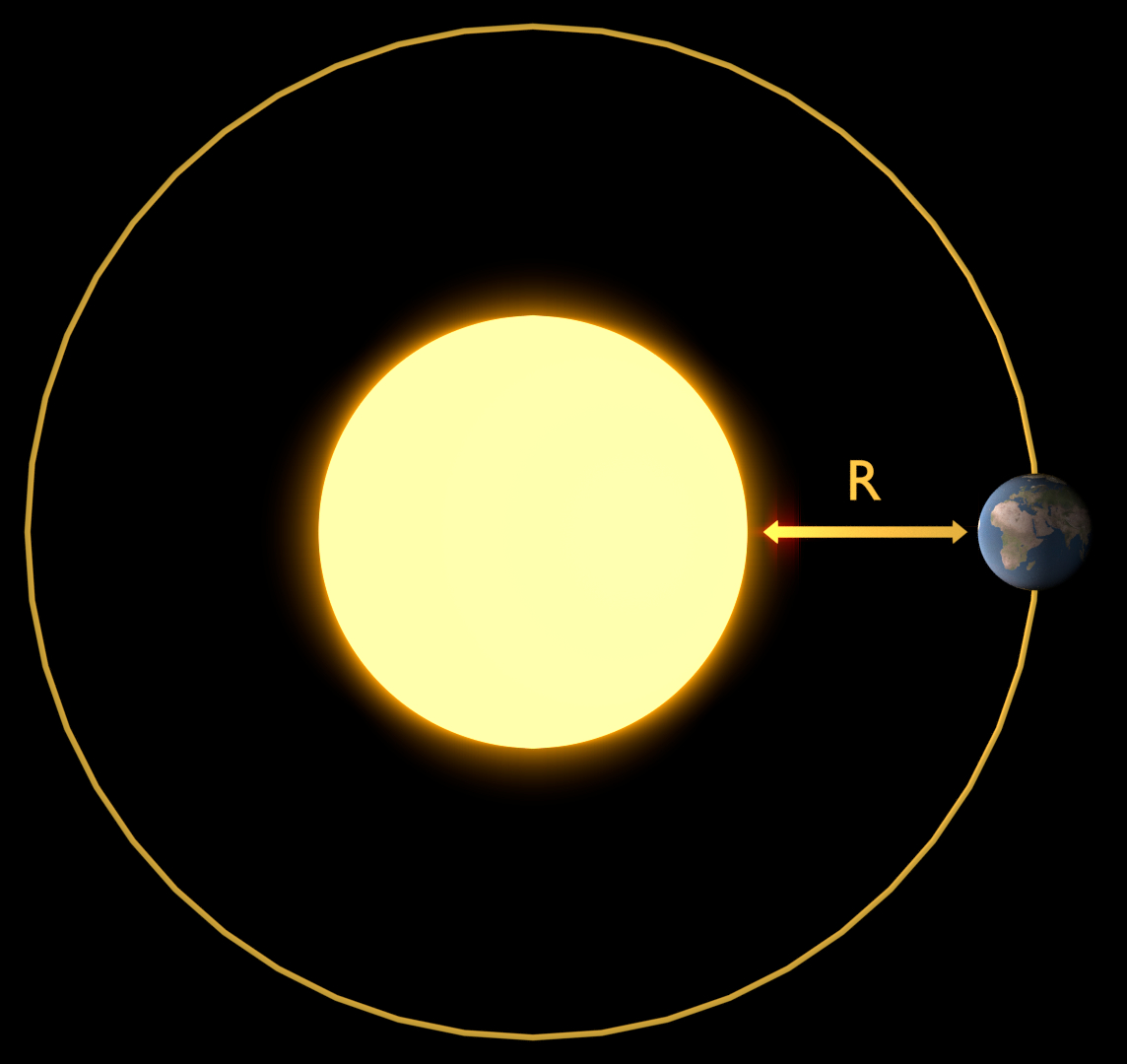In our calculation of $$E_0$$ from $$\Phi_{sol}$$ we need to account for spreading. We do this by dividing $$\Phi_{sol}$$ by the surface area of a sphere with radius R. [image: cc-by Sebastian Mutz]

The sun fuses 4H (H for Hydrogen) to He (Helium), and the resulting mass deficit is resolved by releasing radiation - solar electromagnetic radiation we call $$\Phi_{sol}$$ . $$\Phi_{sol} \approx 3.9 · 10^{26} W$$ .

Sun rays “spread out” into space and radiation becomes less as an inverse square law. Imagine a sphere wrapping around the sun at its centre. This sphere has the radius R, which is the distance between the sun and the Earth. We can account for said spreading by dividing $$\Phi_{sol}$$ by the surface area of a sphere with radius R. You may recall that the surface area (SA) of a sphere is calculated as $$SA = 4·\pi·r^2$$ . We can therefore easily calculate the solar constant from $$\Phi_{sol}$$ as follows:

$\begin{equation} E_0 = \frac { \Phi_{sol} }{ 4·\pi·R^2 } = \frac { 3.9·10^{26} W }{ 4·\pi·(1.5·10^{11} m)^2 } \approx 1380 Wm^{-2} \end{equation}$

Note

What would the solar constant be for Mars?

## Calculating Temperature on Earth¶

We already calculated $$E_0$$ , the radiation intensity hitting the outer layer of Earth. However, in order to calculate global mean temperatures on Earth, we need to know how much of the incoming radiation is absorbed, because only absorbed radiation can do anything on Earth.

Let’s first look at how much radiation is actually intercepted by Earth. Imagine a small globe in a pitch black room that sits next to a light source. When shining the light onto the globe, it casts a shadow behind it. In an idealised setting (without scattering), the shadow will be circular with very sharp edges. The surface area of that circle represents the amount of light that is being intercepted. The surface area of a circle is $$\pi·r^2$$ , where r is its radius. In our case, r is the radius of Earth. We can therefore say that:

$\begin{equation} radiation_{intercepted} = E_0 · \pi · r^2_{earth} \end{equation}$

Note how we went from units of $$Wm^{-2}$$ to $$W$$ by multiplication of $$E_0$$ with an area.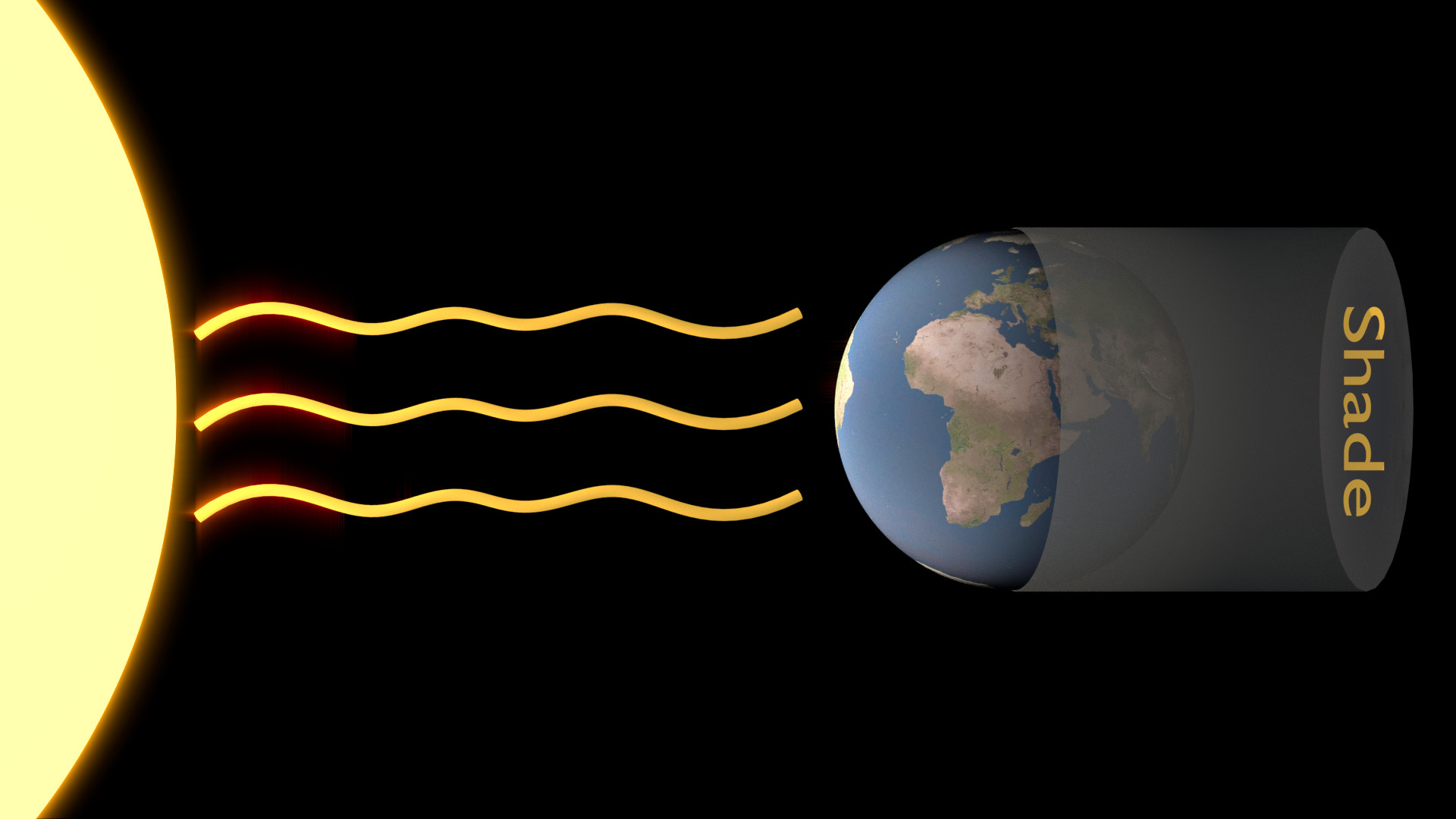The surface area of the circular shade that is hypothetically cast by the Earth as the sun shines onto it from the other side represents the intercepted radiation. [image: cc-by Sebastian Mutz]

Next, we need to take into account that the Earth is not a perfect black body. Earth is fairly reflective and therefore reflects some of the intercepted radiation directly back into space. Note that relfection is not the same as emission. Reflected radiation is not absorbed and re-emitted, but simply “sent” back into space. Albedo ($$\alpha$$ ) is the measure of a planet’s reflectivity. It is a number between 0 and 1. This number is the fraction of incoming radiation that is reflected back into space. Earth has an albedo of ~0.33, which means 33% of incoming radiation is reflected. We can account for it and calculate absorbed radiation as follows:

$\begin{equation} radiation_{absorbed} = E_0 · \pi · r^2_{earth} · (1-\alpha) \end{equation}$

### Earth’s Mean Global Temperature¶

Since our system is in euilibrium, the total amount of radiation absorbed by Earth equals the total amount of radiation emitted Earth. We have previously established that we can calculate the total amount of emitted radiation (per area) using the Stefan-Boltzman equation and the temperature of an object (or planet). In this case, the temperature of the object is the mean global temperature on Earth, i.e. the element we want to calculate! Note that we previously calculated the total absorbed radiation, but the Stephan-Boltzman equation gives us power per area. We therefore have to correct for area before equating the two. To calculate the absorbed radiation per unit area, we can simply divide by the area ( $$\pi·r^2$$ ) again and get:

$\begin{equation} \frac {radiation_{absorbed}}{area} = E_0 · (1-\alpha) \end{equation}$

With the assumption of equilibrium, we can now equate the equations:

$\begin{equation} E_0 · (1-\alpha) = 4 · \sigma · T^4_{earth} \end{equation}$

Note: Since the Stefan-Boltzman equation gives us power per area, we can equate it with the absorbed radiation per area. When uncertain, it helps to check that your units work out!

Finally, we can re-arrange the equation above to solve for $$T_{earth}$$ :

$\begin{equation} T_{earth} = \sqrt { \frac {E_0 · (1-\alpha)} {4 · \sigma}} = \sqrt { \frac {1380 Wm^{-2} · (1-0.33)} {4 · 5.67 · 10^{-8} Wm^{-2}K^{-4} }} \approx 252K \end{equation}$

Note

Do you know the mean global temperature on Earth? How does this compare to our estimate?

## Greenhouse Effect¶

If you converted our estimate of 252K mean global temperature to more commonly used units of °C (subtract 273.15), you may have noticed that our estimate seems a tad cool. You would be right about that, because:

$T_{earth(predicted)} \approx 252K$
$T_{earth(actual)} \approx 288K$

The actual temperature on Earth is about 36K warmer - this makes Earth more habitable for most of the current fauna and flora. The difference comes from one important factor we neglected in our calculation of mean global temperature: greenhouse gases.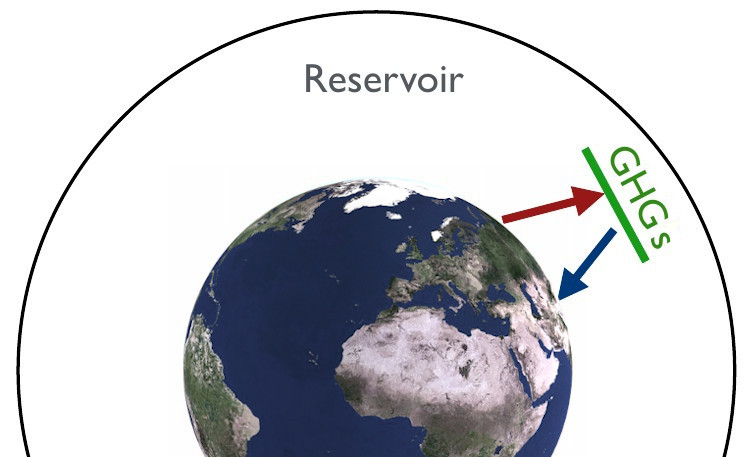Greenhouse gases are the reason Earth is approximately 36K warmer than we would expect the planet to be without an atmosphere (that contains such gases). They absorb radiation and emit some of it back to the planet’s surface.

A greenhouse gas (GHG) is a gas that can absorb and emit infrared radiation. Molecules that have a dipole moment can absorb and emit radiation. Important GHGs on Earth include:

• H2O - a strong absorber in 5.5-7µm range and > 27µm.

• CO2mostly absorbs thermal infrared radiation.

• O3strongly absorbs radiation in the UV spectrum.

## Atmospheric Transmissivity and Opacity¶

Not all wavelengths of radiation are able to pass through an atmosphere equally easily. For some wavelenghts, an atmosphere may be opaque, meaning radiation on of those wavelengths are not able to pass through the atmosphere and reach the planet’s surface. Transmissivity describes this ability. For example, the atmosphere is transparent for radiation in the visible light spectrum, thus allowing transmission in that part of the electromagnetic spectrum.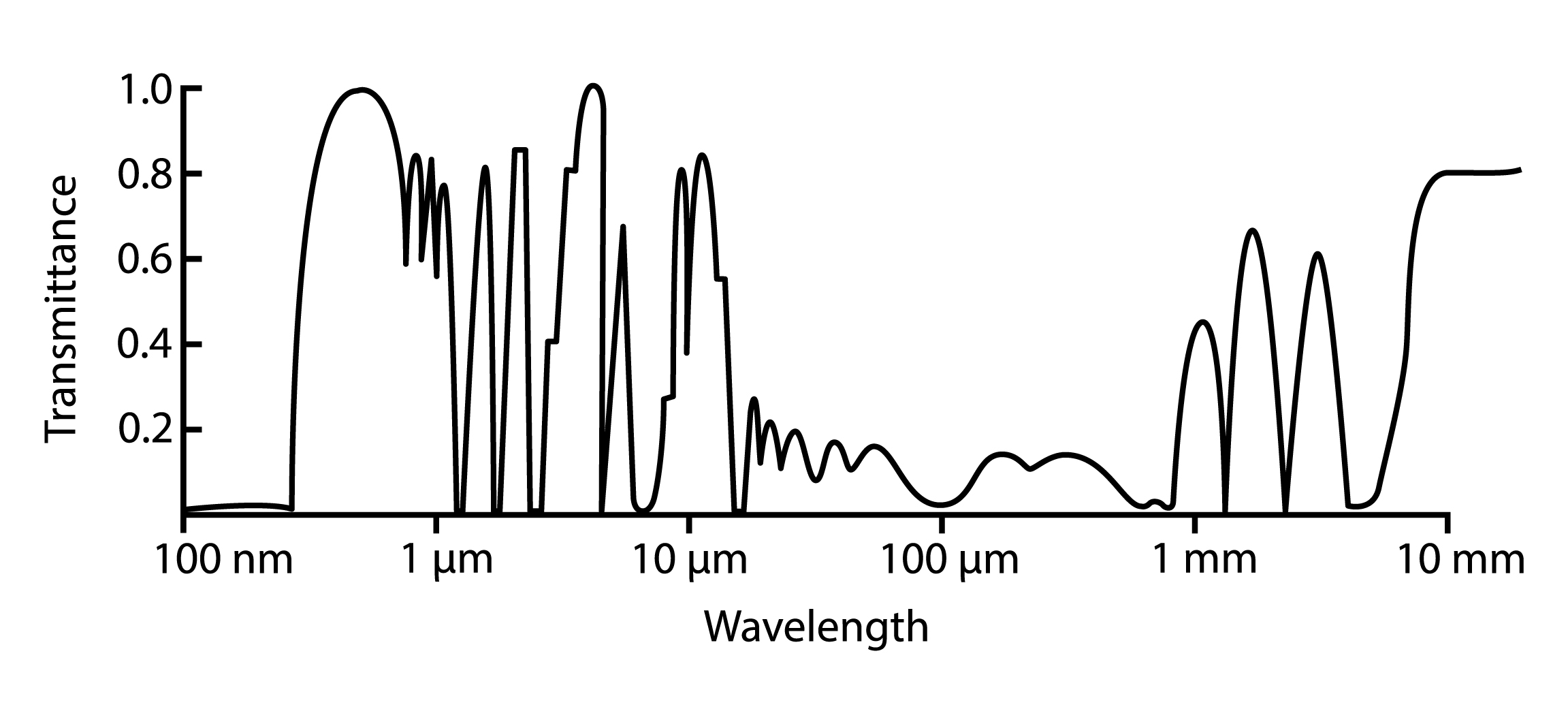The atmosphere is more transparent for some wavelengths than for others. [image: cc-by Lisa Rauschenbach]

Note

Have a closer look at the transmission figure. Do you know where radiowaves fit? Does it make sense to use radio waves for long distance transmission given what you see on this figure?

Note

On what part of the electromagnetic spectrum does Earth’s emission peak? How opaque/transparent is Earth’s atmosphere for radiation emitted by Earth?

$$E_0$$ is the radiation arriving at the top of the atmosphere. However, since the Earth is spherical rather than circular, this is distributed around the globe. Note that the difference between the area of a circle ($$\pi·r^2$$ ) and the area of a sphere ($$4·\pi·r^2$$ ) is a factor of 4, we can account for this by dividing $$E_0$$ by 4. This yields ~ $$345 Wm^{-2}$$ as the radiation per unit area arriving at the top of the atmosphere. (Note that this number is reported differently depending on the book you consult.)